3.5.4 Transition Metals - Variable oxidation states

Specification

 Students should: know that transition elements show variable oxidation states know that Cr3+ and Cr2+ are formed by reduction of Cr2O72- by zinc in acid solution know the redox titration of Fe2+ with MnO4- and Cr2O72- in acid solution be able to perform calculations for this titration and for others when the reductant and its oxidation product are given know the oxidation of Co2+ by air in ammoniacal solution know the oxidation in alkaline solution of Co2+ and Cr3+ by H2O2

Chromium - 4s1 3d5

Chromium forms three common oxidation states in its compounds, +2, +3, +6.

Chromium(VI) - 4s0 3d0

In the +6 state it is a strong oxidising agent, particularly in acidic solution. There are two common series of salts, the chromate(VI) salts and the dichromate(VI) salts. Chromate(VI) is stable in basic solution while dichromate(VI) is stable in acidic solution:

Cr2O72- + OH-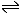2CrO42- + H+

This is not a redox reaction. The equilibrium can be moved from one side to the other by addition of acid or base.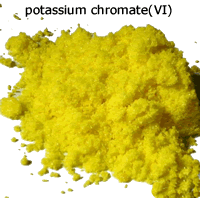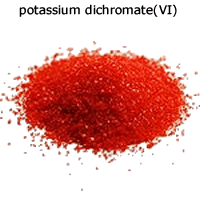The reduction of dichromate ions turns the solution from orange to green as the chromium 3+ ions are formed.

Cr2O72- + 14H+ + 6e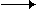2Cr3+ + 7H2O

Potassium dichromate(VI) is the agent of choice for the oxidation of alcohols in organic chemistry.

Acidic potassium dichromate solution reacts with zinc forming chromium 3+ ions. Colour change orange to green.

Cr2O72- + 14H+ + 6e2Cr3+ + 7H2O
3Zn3Zn2+ + 6e
Cr2O72- + 14H+ + 3Zn2Cr3+ + 7H2O + 3Zn2+

and then after further reduction by the zinc, chromium 2+ ions. Colour change green to blue

2Cr3+ + Zn2Cr2+ + Zn2+

Potassium dichromate reacts with iron(II) solutions oxidising them to iron(III).

Cr2O72- + 14H+ + 6e2Cr3+ + 7H2O
6Fe2+6Fe3+ + 6e
Cr2O72- + 14H+ + 6Fe2+2Cr3+ + 7H2O + 6Fe3+

Chromium(III) - 4s0 3d3

Chromium(III) compounds are usually dark green, although chromium(III) chloride is purple when anhydrous. They are formed when potassium dichromate is reduced in acidic solution.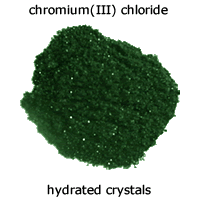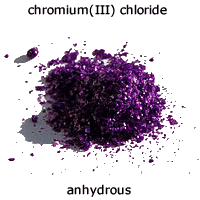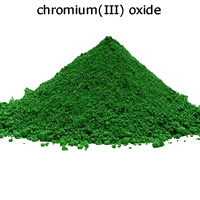Cr2O72- + 14H+ + 3Zn2Cr3+ + 7H2O + 3Zn2+

Chromium(III) salts can be reduced to chromium(II) by strong reducing agents (see below).

Chromium(III) salts can be oxidised by hydrogen peroxide in basic solution:

Firstly the hexaaquachromium(III) complex ions become deprotonated by the strong base to give the hexahydroxychromate(III) complex ion:

[Cr(H2O)6]3+ + 6OH-[Cr(OH)6]3- + 6H2O

This then reacts with hydrogen peroxide to form chromate(VI) ions.

2[Cr(OH)6]3- + 3H2O22CrO42- + 8H2O + 2OH-

Chromium(II) - 4s0 3d4

Chromium(II) compounds are usually blue. They are formed when chromium(III) solutions are reduced by strong reducing agents such as zinc in acidic solution.

2Cr3+ + Zn2Cr2+ + Zn2+top

Manganese - 4s2 3d5

Manganese has five common oxidation states, +2, +3, +4, +6, +7.

Manganese(VI) - 4s0 3d0